Parametric Resonance

Parametric resonance is a resonance phenomenon different from normal resonance and superharmonic resonance because it is an instability phenomenon.

1. The instability

The vertically driven pendulum is the only driven pendulum in the lab which has the same stationary solutions as the undriven pendulum, namely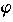= 0 and= 180°. In the undriven case, these solutions are always stable and unstable, respectively. But vertical driving can change stability into instability and vice versa. On this page, mainly the destabilization of the normaly stable equilibrium of the pendulum (i.e.,= 0) will be dicussed. Stabilization of an unstable upside-down pendulum will be mentioned only briefly.

In order to investigate the stability of a fixed point, you have to linearize the equation of motion around a fixed point. For= 0 one gets the (damped) Mathieu equation:.

The same equation holds for= 180° except that02 has a minus sign. Thus, one studies the Mathieu equation for positive as well as negative values of02.Even though the Mathieu equation is a linear differential equation, it can not be solved analytically in terms of standard functions. The reason is that one of the coefficients isn't constant but time-dependent. Fortunatly, the coefficient is periodic in time. This allows to apply the Floquet theorem. It says that in a linear differential equation or a system of linear differential equations there exists a set of fundamental solutions (from which one can build all other solutions) where all solutions can be written in the form(t) = c(t)exp(t), where c(t+1/f) = c(t). The exponentis called Floquet exponent. It isn't uniquely defined because any factor exp(2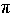it/f) can be either absorbed in c(t) or in exp(t). The solution is stable if the real part of all Floquet exponents are negative. If you are familiar with solid-state physics you will know the Floquet theorem as the Bloch theorem. In fact, the Mathieu equation is just the Schrödinger equation of an electron in a spatially periodic potential where the time variable is interpreted as a space variable. For a fixed value of a (i.e., the strength of the potential), the stable interval of02 corresponds to the bands in the energy spectrum. The unstable intervals are just the band gaps. In the figure the area of instability is shown in blue. It is calculated numerically for a certain value of the damping constant. Note the small area of stability for negative values of02. It denotes the possibility of stabilizing the upside-down pendulum. In the undamped case the areas of stability form tongs (dashed lines) down to zero. That is, an infinitesimal driving amplitude a destabilizes the down-hanging pendulum if the parametric resonance conditionis fulfilled, where n is an integer defining the order of parametric resonance. In case of damping, a driving amplitude a exceeding a critical value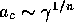is necessary for destabilization.

There is a simple intuitive understanding of the parametric resonance condition. And maybe you already have such intuitive knowledge! Imagine you are on a fair and you want to swing a swing boat (did you ever try?). Standing in the boat you have to go down with your body when reaching a maximum because you want to speed the boat up by putting an additional acceleration to the acceleration of gravity. If you do that near the forward and the backward maximum of oscillation, you are just realizing first-order parametric resonance because you are moving periodically up and down with a frequency just twice the frequency of the swing boat. If you go down only every nth maximum you will have parametric resonance of order n.

The onset of first-order parametric resonance can be approximated analytically very well by the ansatz:This ansatz is a truncated Fourier series of the periodic function c(t) of the Floquet theorem. It neither decays nor grows. Thus the amplitude a of driving has to be just the critical one ac. Put this ansatz into (1) and neglect the terms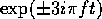. Now, gather all terms having the factorand you will getThese two equations have a nontrivial solution for c+ and c- only if

 (3)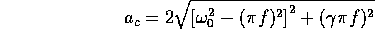This instability threshold has a minimum just at the parametric resonance condition f =0/(plus a correction of second order in). The minimum reads ac=2f.

2. Parametrically excited oscillations

 g = 9.81 m/sec2,  l = 1 m,= 0.1 sec-1,  A = 0.07 m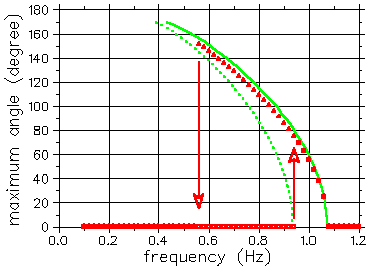In parametric resonance the amplitude of the unstable solution grows exponentially to infinity. Damping does not help to saturate this growth contrary to normal resonance caused by an additive driving force. Thus, the nonlinearities in the parametrically driven pendulum are necessary for saturation. This saturation is caused by the fact that nonlinear oscillators have in general an amplitude-dependent eigenfrequency. The growth of the parametrically excited oscillation will shift the eigenfrequency out of resonance. Thus a good approximation of the amplitudemax of the oscillation excited in first-order parametric resonance would be Eq. (3) where0 is replaced byres(max). The figure compares this approximation (green curves) with numerical solutions (red symbols). The approximation is indeed marvelous! Formax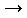0 the green curves end at the boundaries of the instability interval. In the language of nonlinear dynamics they mark supercritical and subcritical period doubling bifurcations. Thus one curve (dotted line) corresponds to an unstable solution. It is inbetween the stable down-hanging equilibrium and the stable oscillation. This bistability is responsible for hysteresis. Again as for nonlinear resonance a foldover effect has bend the resonance curves.

 QUESTIONS worth to think about: Does parametric resonance also appear for other driven pendula in the Pendulum Lab? Imagine a media where waves (e.g. sound waves) can propagate. What might happen if the speed of wave is modulated periodically?

© 1998 Franz-Josef Elmer,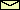Franz-Josef doht Elmer aht unibas doht ch, last modified Monday, July 20, 1998.